# 为什么学习 HashMap 源码？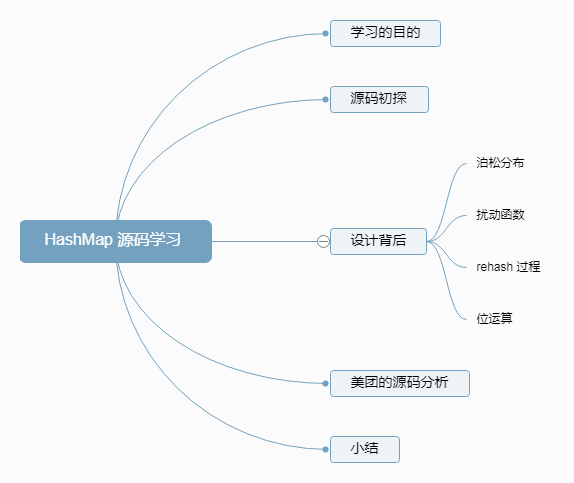（1）让读者理解 HashMap 的设计思想，知道 rehash 的过程。下一节我们将自己实现一个 HashMap

（2）为什么要自己实现 HashMap?

# HashMap 源码

HashMap 是平时使用到非常多的一个集合类，感觉有必要深入学习一下。

## java 版本

\$ java -version
java version "1.8.0_91"
Java(TM) SE Runtime Environment (build 1.8.0_91-b14)
Java HotSpot(TM) 64-Bit Server VM (build 25.91-b14, mixed mode)


## 对于当前类的官方说明

HashMap实例有两个影响其性能的参数: 初始容量和负载因子

Map m = Collections.synchronizedMap(new HashMap(...));


## 其他基础信息

1. 这个类是Java集合框架的成员。

2. @since 1.2

3. java.util 包下

# 源码初探

## 接口

public class HashMap<K,V> extends AbstractMap<K,V>
implements Map<K,V>, Cloneable, Serializable {}


# 常量定义

## 默认初始化容量

/**
* The default initial capacity - MUST be a power of two.
*/
static final int DEFAULT_INITIAL_CAPACITY = 1 << 4; // aka 16

• 为什么不直接使用 16？

1. 为了避免使用魔法数字，使得常量定义本身就具有自我解释的含义。

2. 强调这个数必须是 2 的幂。

• 为什么要是 2 的幂？

final Node<K,V> getNode(int hash, Object key) {
Node<K,V>[] tab; Node<K,V> first, e; int n; K k;
if ((tab = table) != null && (n = tab.length) > 0 &&
(first = tab[(n - 1) & hash]) != null) { /// <-- bitwise 'AND' here
...


## 最大容量

/**
* The maximum capacity, used if a higher value is implicitly specified
* by either of the constructors with arguments.
* MUST be a power of two <= 1<<30.
*/
static final int MAXIMUM_CAPACITY = 1 << 30;

• 为什么是 1 « 30？

## 负载因子

/**
* The load factor used when none specified in constructor.
*/
static final float DEFAULT_LOAD_FACTOR = 0.75f;

• 为什么是 0.75，不是 0.8 或者 0.6

Because TreeNodes are about twice the size of regular nodes, we
use them only when bins contain enough nodes to warrant use
(see TREEIFY_THRESHOLD). And when they become too small (due to
removal or resizing) they are converted back to plain bins.  In
usages with well-distributed user hashCodes, tree bins are
rarely used.  Ideally, under random hashCodes, the frequency of
nodes in bins follows a Poisson distribution
(http://en.wikipedia.org/wiki/Poisson_distribution) with a
parameter of about 0.5 on average for the default resizing
threshold of 0.75, although with a large variance because of
resizing granularity. Ignoring variance, the expected
occurrences of list size k are (exp(-0.5) * pow(0.5, k) /
factorial(k)). The first values are:

0:    0.60653066
1:    0.30326533
2:    0.07581633
3:    0.01263606
4:    0.00157952
5:    0.00015795
6:    0.00001316
7:    0.00000094
8:    0.00000006
more: less than 1 in ten million


Poisson distribution —— 泊松分布

## 阈值

/**
* The bin count threshold for using a tree rather than list for a
* bin.  Bins are converted to trees when adding an element to a
* bin with at least this many nodes. The value must be greater
* than 2 and should be at least 8 to mesh with assumptions in
* tree removal about conversion back to plain bins upon
* shrinkage.
*/
static final int TREEIFY_THRESHOLD = 8;

/**
* The bin count threshold for untreeifying a (split) bin during a
* resize operation. Should be less than TREEIFY_THRESHOLD, and at
* most 6 to mesh with shrinkage detection under removal.
*/
static final int UNTREEIFY_THRESHOLD = 6;

/**
* The smallest table capacity for which bins may be treeified.
* (Otherwise the table is resized if too many nodes in a bin.)
* Should be at least 4 * TREEIFY_THRESHOLD to avoid conflicts
* between resizing and treeification thresholds.
*/
static final int MIN_TREEIFY_CAPACITY = 64;


# Node

## 源码

• Node.java

/**
* Basic hash bin node, used for most entries.  (See below for
* TreeNode subclass, and in LinkedHashMap for its Entry subclass.)
*/
static class Node<K,V> implements Map.Entry<K,V> {
final int hash;
final K key;
V value;
Node<K,V> next;
Node(int hash, K key, V value, Node<K,V> next) {
this.hash = hash;
this.key = key;
this.value = value;
this.next = next;
}
public final K getKey()        { return key; }
public final V getValue()      { return value; }
public final String toString() { return key + "=" + value; }
public final int hashCode() {
return Objects.hashCode(key) ^ Objects.hashCode(value);
}
public final V setValue(V newValue) {
V oldValue = value;
value = newValue;
return oldValue;
}
public final boolean equals(Object o) {
// 快速判断
if (o == this)
return true;

// 类型判断
if (o instanceof Map.Entry) {
Map.Entry<?,?> e = (Map.Entry<?,?>)o;
if (Objects.equals(key, e.getKey()) &&
Objects.equals(value, e.getValue()))
return true;
}
return false;
}
}


## 个人理解

final int hash; // hash 值
final K key;    // key
V value;    // value 值
Node<K,V> next; // 下一个元素结点


### hash 值的算法

hash 算法如下。

Objects.hashCode(key) ^ Objects.hashCode(value);


public static int hashCode(Object o) {
return o != null ? o.hashCode() : 0;
}


# 静态工具类

## hash

static final int hash(Object key) {
int h;
return (key == null) ? 0 : (h = key.hashCode()) ^ (h >>> 16);
}


### 为什么这么设计？

• jdk8 自带解释

(已知的例子中有一组浮点键，它们在小表中保存连续的整数。)

• 知乎的解释

HashMap扩容之前的数组初始大小才16。所以这个散列值是不能直接拿来用的。

putVal 函数源码

final V putVal(int hash, K key, V value, boolean onlyIfAbsent,
boolean evict) {
Node<K,V>[] tab; Node<K,V> p; int n, i;
if ((tab = table) == null || (n = tab.length) == 0)
n = (tab = resize()).length;
if ((p = tab[i = (n - 1) & hash]) == null)
tab[i] = newNode(hash, key, value, null);
//...
}


    10100101 11000100 00100101
&	00000000 00000000 00001111
-------------------------------
00000000 00000000 00000101    //高位全部归零，只保留末四位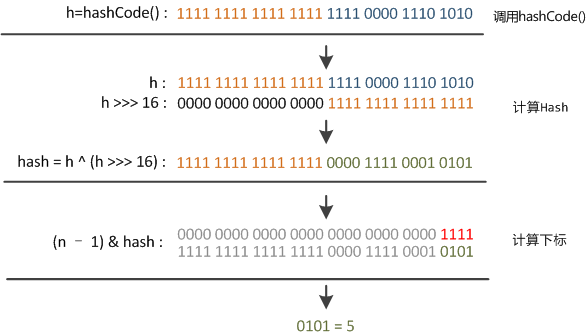## comparable class

• comparableClassFor()

ps: 这个方法很有借鉴意义，可以做简单的拓展。我们可以获取任意接口泛型中的类型。

static Class<?> comparableClassFor(Object x) {
if (x instanceof Comparable) {
Class<?> c; Type[] ts, as; Type t; ParameterizedType p;
if ((c = x.getClass()) == String.class) // bypass checks
return c;
if ((ts = c.getGenericInterfaces()) != null) {
for (int i = 0; i < ts.length; ++i) {
if (((t = ts[i]) instanceof ParameterizedType) &&
((p = (ParameterizedType)t).getRawType() ==
Comparable.class) &&
(as = p.getActualTypeArguments()) != null &&
as.length == 1 && as == c) // type arg is c
return c;
}
}
}
return null;
}


## compareComparables()

@SuppressWarnings({"rawtypes","unchecked"}) // for cast to Comparable
static int compareComparables(Class<?> kc, Object k, Object x) {
return (x == null || x.getClass() != kc ? 0 :
((Comparable)k).compareTo(x));
}


## tableSizeFor

static final int tableSizeFor(int cap) {
int n = cap - 1;
n |= n >>> 1;
n |= n >>> 2;
n |= n >>> 4;
n |= n >>> 8;
n |= n >>> 16;
return (n < 0) ? 1 : (n >= MAXIMUM_CAPACITY) ? MAXIMUM_CAPACITY : n + 1;
}

• 被调用处
public HashMap(int initialCapacity, float loadFactor) {
// check...
this.threshold = tableSizeFor(initialCapacity);
}

• 感想

emmm….为什么要这么写？性能吗？

### 简单分析

• 为什么要 -1

int n = cap - 1;

• 第一次位运算

n |= n >>> 1;

### 实例

表达式                       二进制
------------------------------------------------------

initialCapacity = 10;
int n = 9;                  0000 1001
------------------------------------------------------

n |= n >>> 1;               0000 1001
0000 0100   (右移1位) 或运算
= 0000 1101
------------------------------------------------------

n |= n >>> 2;               0000 1101
0000 0011   (右移2位) 或运算
= 0000 1111
------------------------------------------------------

n |= n >>> 4;               0000 1111
0000 0000   (右移4位) 或运算
= 0000 1111
------------------------------------------------------

n |= n >>> 8;               0000 1111
0000 0000   (右移8位) 或运算
= 0000 1111
------------------------------------------------------

n |= n >>> 16;              0000 1111
0000 0000   (右移16位) 或运算
= 0000 1111
------------------------------------------------------

n = n+1;                    0001 0000    结果：2^4 = 16;


# put() 解释

## 流程图解

HashMap的put方法执行过程可以通过下图来理解，自己有兴趣可以去对比源码更清楚地研究学习。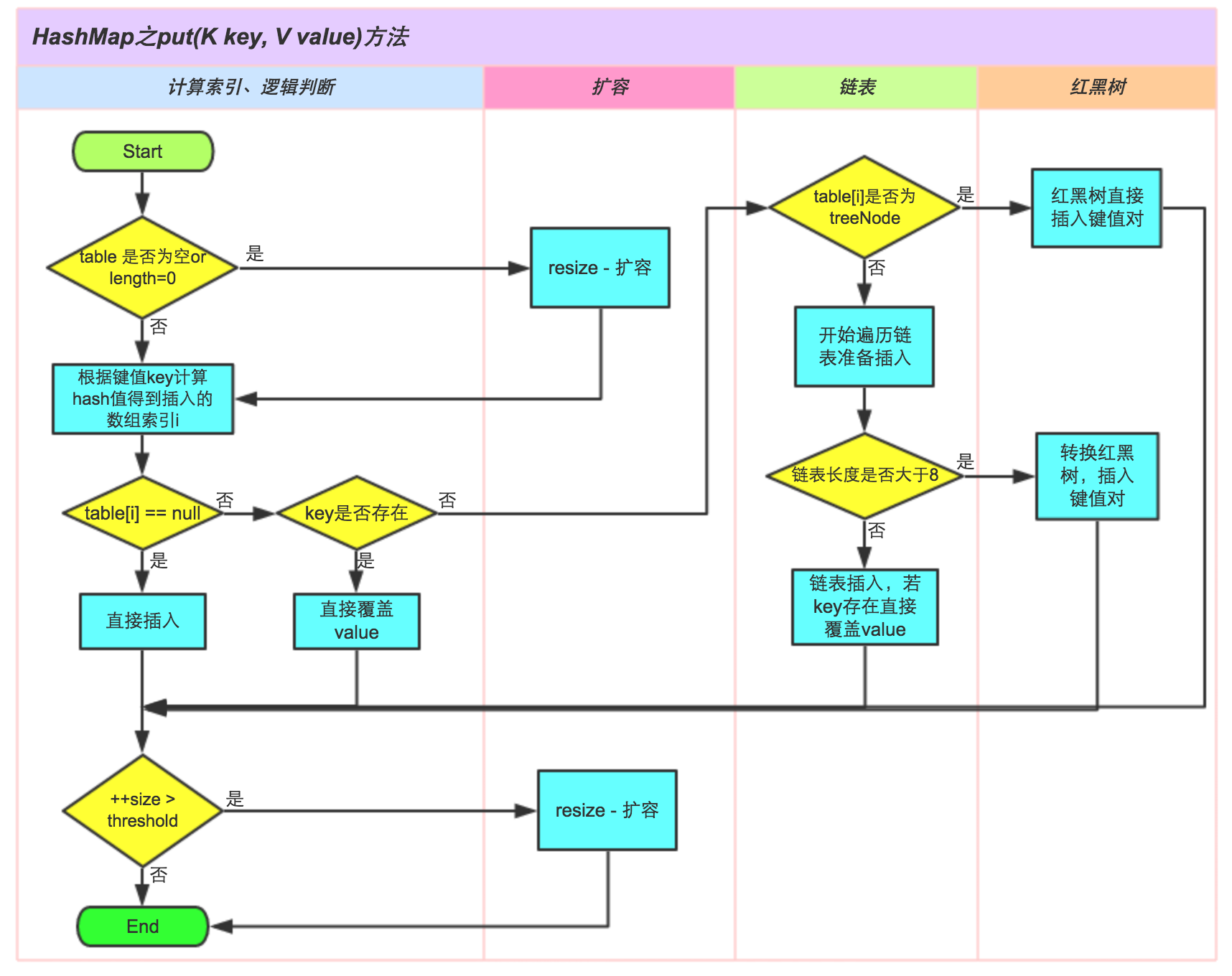①.判断键值对数组table[i]是否为空或为null，否则执行resize()进行扩容；

②.根据键值key计算hash值得到插入的数组索引i，如果table[i]==null，直接新建节点添加，转向⑥，如果table[i]不为空，转向③；

③.判断table[i]的首个元素是否和key一样，如果相同直接覆盖value，否则转向④，这里的相同指的是hashCode以及equals；

④.判断table[i] 是否为treeNode，即table[i] 是否是红黑树，如果是红黑树，则直接在树中插入键值对，否则转向⑤；

⑤.遍历table[i]，判断链表长度是否大于8，大于8的话把链表转换为红黑树，在红黑树中执行插入操作，否则进行链表的插入操作；遍历过程中若发现key已经存在直接覆盖value即可；

⑥.插入成功后，判断实际存在的键值对数量size是否超多了最大容量threshold，如果超过，进行扩容。

## 方法源码

public V put(K key, V value) {
return putVal(hash(key), key, value, false, true);
}
/**
* Implements Map.put and related methods
*
* @param hash hash for key
* @param key the key
* @param value the value to put
* @param onlyIfAbsent if true, don't change existing value
* @param evict if false, the table is in creation mode.
* @return previous value, or null if none
*/
final V putVal(int hash, K key, V value, boolean onlyIfAbsent,
boolean evict) {
Node<K,V>[] tab; Node<K,V> p; int n, i;
if ((tab = table) == null || (n = tab.length) == 0)
n = (tab = resize()).length;
if ((p = tab[i = (n - 1) & hash]) == null)
tab[i] = newNode(hash, key, value, null);
else {
Node<K,V> e; K k;
if (p.hash == hash &&
((k = p.key) == key || (key != null && key.equals(k))))
e = p;
else if (p instanceof TreeNode)
e = ((TreeNode<K,V>)p).putTreeVal(this, tab, hash, key, value);
else {
for (int binCount = 0; ; ++binCount) {
if ((e = p.next) == null) {
p.next = newNode(hash, key, value, null);
if (binCount >= TREEIFY_THRESHOLD - 1) // -1 for 1st
treeifyBin(tab, hash);
break;
}
if (e.hash == hash &&
((k = e.key) == key || (key != null && key.equals(k))))
break;
p = e;
}
}
if (e != null) { // existing mapping for key
V oldValue = e.value;
if (!onlyIfAbsent || oldValue == null)
e.value = value;
afterNodeAccess(e);
return oldValue;
}
}
++modCount;
if (++size > threshold)
resize();
afterNodeInsertion(evict);
return null;
}


# 扩容机制

## JDK7 源码

void resize(int newCapacity) {   //传入新的容量
Entry[] oldTable = table;    //引用扩容前的Entry数组
int oldCapacity = oldTable.length;
if (oldCapacity == MAXIMUM_CAPACITY) {  //扩容前的数组大小如果已经达到最大(2^30)了
threshold = Integer.MAX_VALUE; //修改阈值为int的最大值(2^31-1)，这样以后就不会扩容了
return;
}

Entry[] newTable = new Entry[newCapacity];  //初始化一个新的Entry数组
transfer(newTable);                         //！！将数据转移到新的Entry数组里
table = newTable;                           //HashMap的table属性引用新的Entry数组
}


void transfer(Entry[] newTable) {
Entry[] src = table;                   //src引用了旧的Entry数组
int newCapacity = newTable.length;
for (int j = 0; j < src.length; j++) { //遍历旧的Entry数组
Entry<K,V> e = src[j];             //取得旧Entry数组的每个元素
if (e != null) {
src[j] = null;//释放旧Entry数组的对象引用（for循环后，旧的Entry数组不再引用任何对象）
do {
Entry<K,V> next = e.next;
int i = indexFor(e.hash, newCapacity); //！！重新计算每个元素在数组中的位置
e.next = newTable[i]; //标记
newTable[i] = e;      //将元素放在数组上
e = next;             //访问下一个Entry链上的元素
} while (e != null);
}
}
}


newTable[i]的引用赋给了e.next，也就是使用了单链表的头插入方式，同一位置上新元素总会被放在链表的头部位置；

## 案例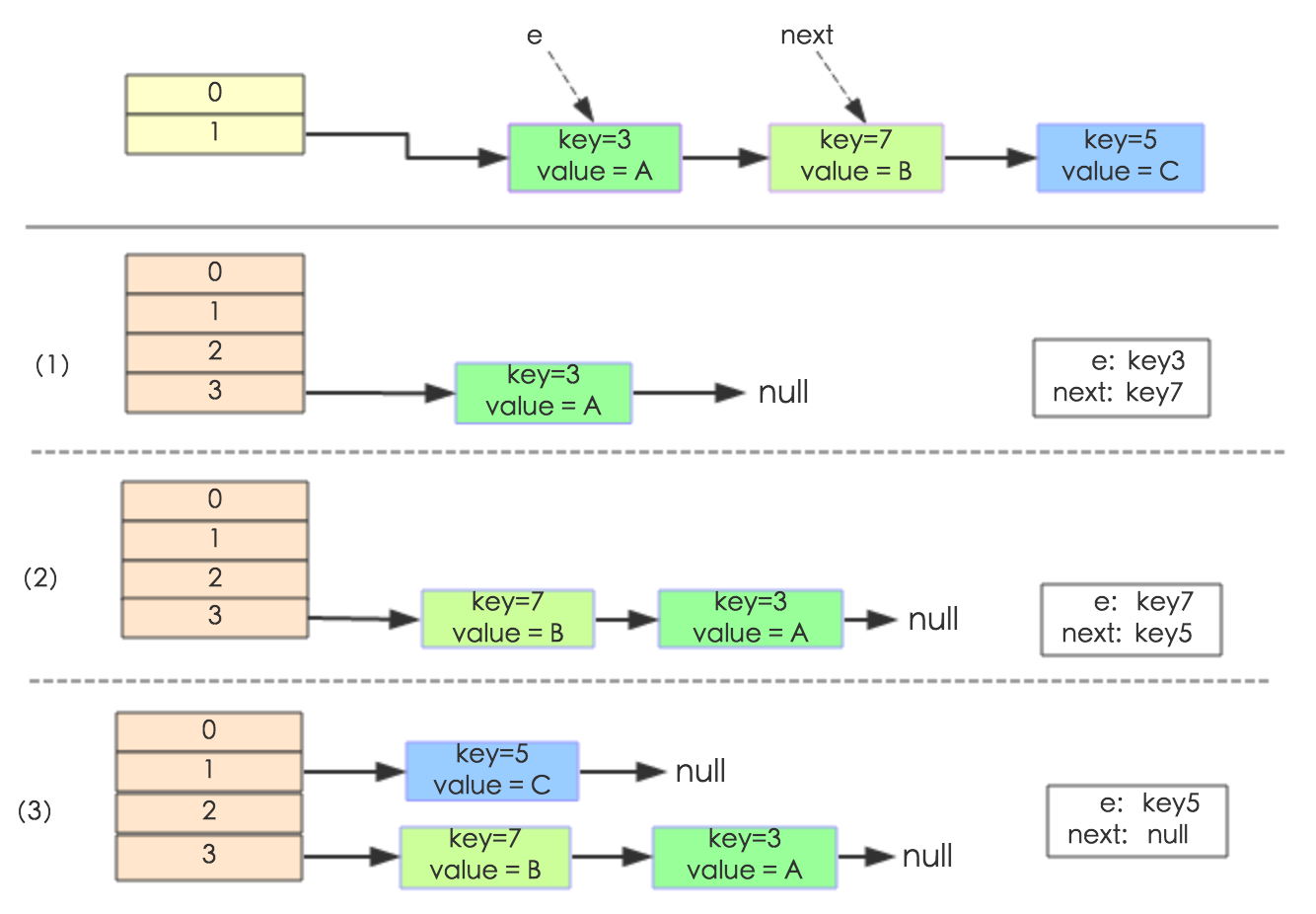## Jdk8 优化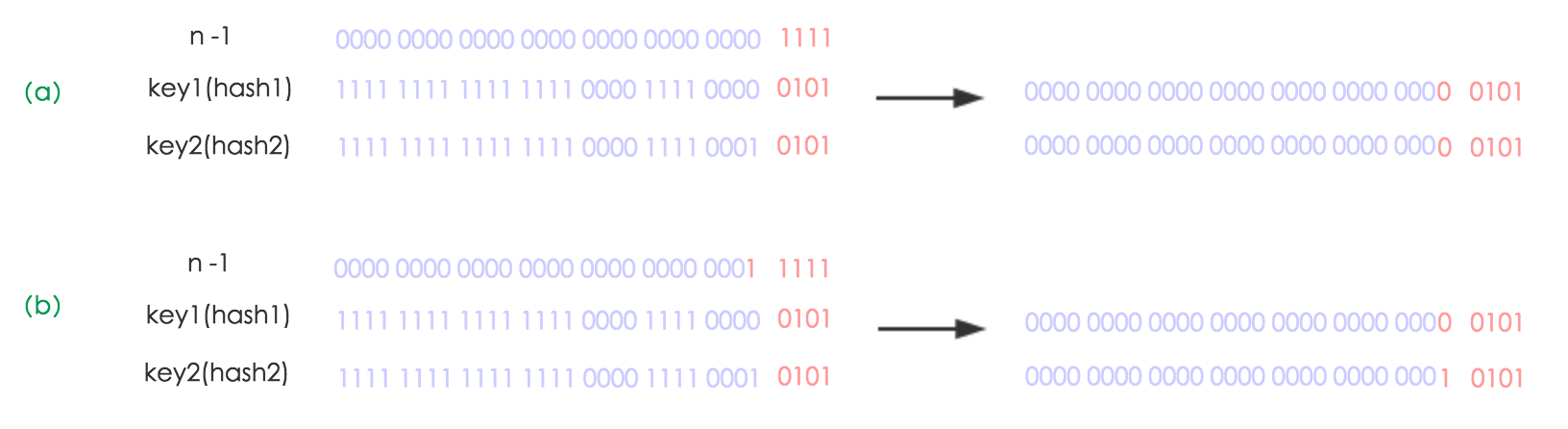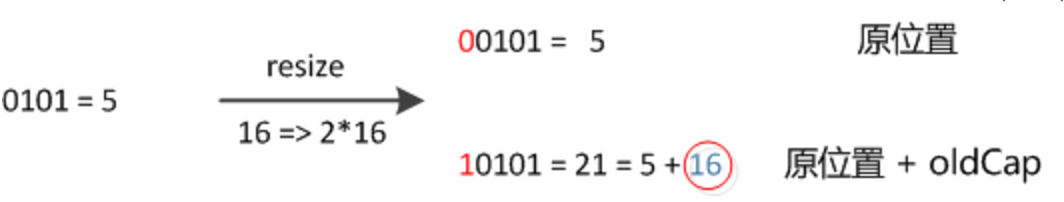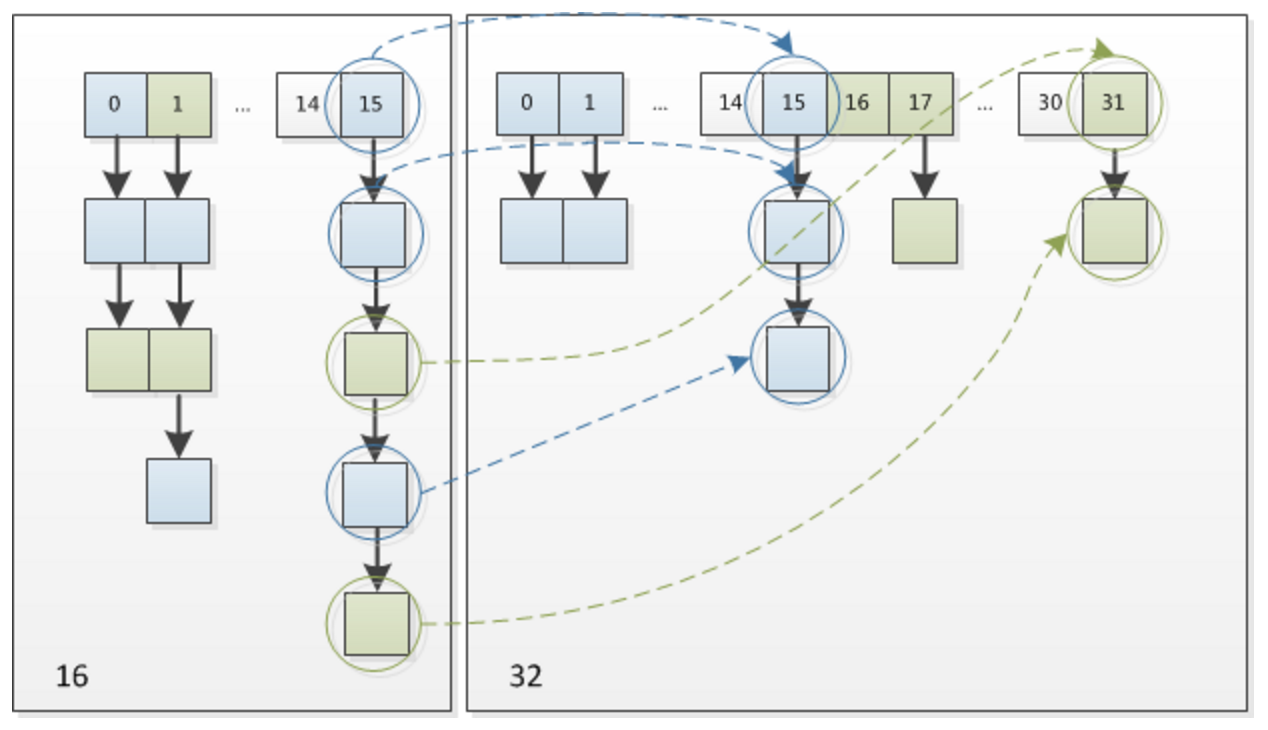## JDK8 源码

/**
* Initializes or doubles table size.  If null, allocates in
* accord with initial capacity target held in field threshold.
* Otherwise, because we are using power-of-two expansion, the
* elements from each bin must either stay at same index, or move
* with a power of two offset in the new table.
*
* @return the table
*/
final Node<K,V>[] resize() {
Node<K,V>[] oldTab = table;
int oldCap = (oldTab == null) ? 0 : oldTab.length;
int oldThr = threshold;
int newCap, newThr = 0;
if (oldCap > 0) {
if (oldCap >= MAXIMUM_CAPACITY) {
threshold = Integer.MAX_VALUE;
return oldTab;
}
else if ((newCap = oldCap << 1) < MAXIMUM_CAPACITY &&
oldCap >= DEFAULT_INITIAL_CAPACITY)
newThr = oldThr << 1; // double threshold
}
else if (oldThr > 0) // initial capacity was placed in threshold
newCap = oldThr;
else {               // zero initial threshold signifies using defaults
newCap = DEFAULT_INITIAL_CAPACITY;
}
if (newThr == 0) {
float ft = (float)newCap * loadFactor;
newThr = (newCap < MAXIMUM_CAPACITY && ft < (float)MAXIMUM_CAPACITY ?
(int)ft : Integer.MAX_VALUE);
}
threshold = newThr;
@SuppressWarnings({"rawtypes","unchecked"})
Node<K,V>[] newTab = (Node<K,V>[])new Node[newCap];
table = newTab;
if (oldTab != null) {
for (int j = 0; j < oldCap; ++j) {
Node<K,V> e;
if ((e = oldTab[j]) != null) {
oldTab[j] = null;
if (e.next == null)
newTab[e.hash & (newCap - 1)] = e;
else if (e instanceof TreeNode)
((TreeNode<K,V>)e).split(this, newTab, j, oldCap);
else { // preserve order
Node<K,V> loHead = null, loTail = null;
Node<K,V> hiHead = null, hiTail = null;
Node<K,V> next;
do {
next = e.next;
if ((e.hash & oldCap) == 0) {
if (loTail == null)
else
loTail.next = e;
loTail = e;
}
else {
if (hiTail == null)
else
hiTail.next = e;
hiTail = e;
}
} while ((e = next) != null);
if (loTail != null) {
loTail.next = null;
}
if (hiTail != null) {
hiTail.next = null;
}
}
}
}
}
return newTab;
}


# 拓展阅读

java transient 关键字

# 参考资料

oracle jdk8 doc

## 常量定义

why-is-the-maximum-capacity-of-a-java-hashmap-130-and-not-131

• 负载因子

https://www.cnblogs.com/a294098789/p/5323032.html

https://blog.csdn.net/wenyiqingnianiii/article/details/52204136

## 静态工具方法

• hash

why-return-h-key-hashcode-h-16-other-than-key-hashcode

JDK 源码中 HashMap 的 hash 方法原理是什么？

hash 的生成策略

• tableSizeFor

Java8 HashMap之tableSizeFor

## map 源码分析

Java8系列之重新认识HashMap

Java 8系列之重新认识HashMap